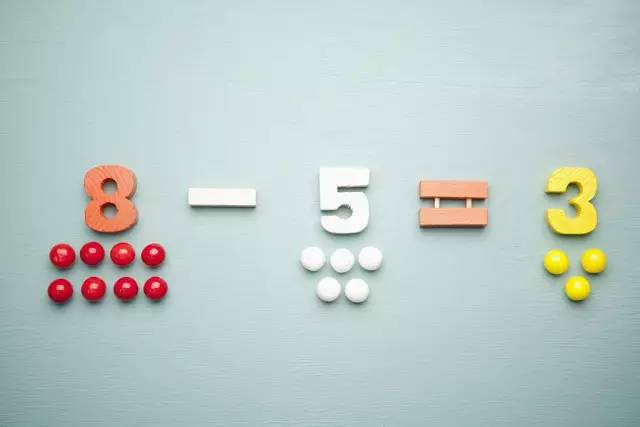21世纪教育网，面向全国的中小学学教师、家长交流平台

# 2021年小学数学必背公式大全

日期:2021-10-22 09:28 阅读:
在小学数学的学习中，公式是学习的重点。下面小编整理了小学数学必背公式大全，供大家参考。## 一、关系表达式

1、每份数×份数=总数总数÷每份数=份数总数÷份数=每份数

2、 1倍数×倍数=几倍数几倍数÷1倍数=倍数几倍数÷倍数=1倍数 3、速度×时间=路程路程÷速度=时间路程÷时间=速度

4、单价×数量=总价总价÷单价=数量总价÷数量=单价

5、工作效率×工作时间=工作总量工作总量÷工作效率=工作时间工作总量÷工作时间=工作效率

6、加数+加数=和和-一个加数=另一个加数

7、被减数-减数=差被减数-差=减数差+减数=被减数

8、因数×因数=积积÷一个因数=另一个因数

9、被除数÷除数=商被除数÷商=除数商×除数=被除数

## 二、单位间进率

1公里=1千米 1千米=1000 米

1米=10分米 1分米=10厘米 1厘米=10毫米

1平方米=100平方分米 1平方分米=100平方厘米 1平方厘米=100平方毫米

1立方米=1000立方分米 1立方分米=1000立方厘米 1立方厘米=1000立方毫米

1吨=1000千克 1千克= 1000克= 1公斤= 1市斤

1公顷=10000平方米 1亩=666.666平方米

1升=1立方分米=1000毫升 1毫升=1立方厘米

## 三、几何形体周长面积体积计算公式

1、长方形的周长=(长+宽)×2 C=(a+b)×2

2、正方形的周长=边长×4 C=4a

3、长方形的面积=长×宽 S=ab

4、正方形的面积=边长×边长 S=a.a= a

5、三角形的面积=底×高÷2 S=ah÷2

6、平行四边形的面积=底×高 S=ah

7、梯形的面积=(上底+下底)×高÷2 S=(a+b)h÷2

8、直径=半径×2 d=2r 半径=直径÷2 r= d÷2

9、圆的周长=圆周率×直径=圆周率×半径×2 c=πd =2πr

10、圆的面积=圆周率×半径×半径

11、长方体的表面积=(长×宽+长×高+宽×高) ×2 公式：S=(a×b+a×c+b×c)×2

12、长方体的体积=长×宽×高公式：V = abh

13、正方体的表面积=棱长×棱长×6 公式： S=6a2

14、长方体(或正方体)的体积=底面积×高公式：V = abh
Tags：2021年,小学,数学,必背,公式